# Prime numbers + natural numbers - math problems

#### Number of problems found: 141

• Prime factorsFactor the number 6600 into the product of prime numbers.
• Sum of two primesChristian Goldbach, a mathematician, found out that every even number greater than 2 can be expressed as a sum of two prime numbers. Write or express 2018 as a sum of two prime numbers.
• Primes 2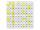Which prime numbers is number 2025 divisible?
• Prime factorsWrite 98 as a product of prime factors
• Prime divisorsFind 2/3 of the sum's ratio and the product of all prime divisors of the number 120.
• Count of roots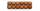How many solutions has equation x. y = 7757 with two unknowns on the set of natural numbers?
• Six-digit primesFind all six-digit prime numbers that contain each one of digits 1,2,4,5,7, and 8 just once. How many are they?
• Divisible by nineHow many three-digit natural numbers in total are divisible without a remainder by the number 9?
• Twenty-fiveHow many are three-digit natural numbers divisible by 25?
• RectanglesHow many rectangles with area 8713 cm2 whose sides are natural numbers are?
• Year 2018The product of the three positive numbers is 2018. What are the numbers?
• Lcm of three numbersWhat is the Lcm of 120 15 and 5
• Reminder and quotientThere are given the number C = 281, D = 201. Find the highest natural number S so that the C:S, D:S are with the remainder of 1,
• Lcm 2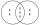Create the smallest possible number that is divisible by numbers 5,8,9,4,3
• MO C–I–1 2018An unknown number is divisible by just four numbers from the set {6, 15, 20, 21, 70}. Determine which ones.
• Common divisorsFind all common divisors of numbers 30 and 45.
• Z7-I-4 stars 4949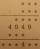Write instead of stars digits so the next write of product of the two numbers to be valid: ∗ ∗ ∗ · ∗ ∗ ∗ ∗ ∗ ∗ ∗ 4 9 4 9 ∗ ∗ ∗ ∗ ∗ ∗ 4 ∗ ∗
• There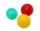There are two numbers on the screen - one in the blue and the other in the red box. In the beginning, both numbers are the same. With each beep, both numbers increase - by 1 in the blue field and by 3 in the red field. At one point, the number 49 appears
• John SmithJohn Smith is reading a 435-page novel. If he reads the same number of pages per day and the number is a prime number greater than 10, how many pages per day will he read?
• NumbersDetermine the number of all positive integers less than 4183444 if each is divisible by 29, 7, 17. What is its sum?

Do you have an interesting mathematical word problem that you can't solve it? Submit a math problem, and we can try to solve it.

We will send a solution to your e-mail address. Solved examples are also published here. Please enter the e-mail correctly and check whether you don't have a full mailbox.

Please do not submit problems from current active competitions such as Mathematical Olympiad, correspondence seminars etc...

Prime numbers - math problems. Natural numbers - math problems.